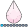NKormanik
Barite | Level 11

## General Optimization Question for Starters

I'm trying to come up with a combination of variables that seem optimal, or 'best.'

Please note the following part of a dataset:

``````Combo_Plus	N	Slope_5040x	Slope_5050x	_50401	_50402	_50403	_50404	_50405	_50408	_50501	_50502	_50503	_50504	_50505	_50508
_22301_1  _22304_6	134	-0.781955	1.16525	-0.39263	-0.73782	-1.67705	-1.67705	-1.95345	-6.17685	-0.39263	0.17215	3.29095	4.62515	4.99405	7.50648
_22301_1  _22304_6	111	-0.781955	1.16525	-0.39263	-0.73782	-1.67705	-1.67705	-1.95345	-6.17685	-0.39263	0.17215	3.29095	4.62515	4.99405	7.50648
_20404_5  _22602_0	93	-0.405838	1.16482	-0.43710	-1.97530	-2.09233	-2.09233	-2.09233	-3.91848	-0.43710	0.85453	2.63358	5.12683	5.96438	7.35008
_20404_5  _22602_0	54	-0.405838	1.16482	-0.43710	-1.97530	-2.09233	-2.09233	-2.09233	-3.91848	-0.43710	0.85453	2.63358	5.12683	5.96438	7.35008
_21704_1  _21801a_5	72	-0.028845	1.16109	0.16725	-0.00315	-0.09027	-0.09027	-0.09027	-0.09027	0.16725	1.15110	2.57457	3.77747	4.88880	8.20720
_21704_1  _21801a_5	166	-0.028845	1.16109	0.16725	-0.00315	-0.09027	-0.09027	-0.09027	-0.09027	0.16725	1.15110	2.57457	3.77747	4.88880	8.20720
_21502_0  _22403_6	81	-0.462939	1.16064	1.47176	0.86975	0.09590	-0.93499	-1.34712	-1.72389	1.47176	2.92255	3.53350	5.25229	7.11890	9.37875
_21502_0  _22403_6	127	-0.462939	1.16064	1.47176	0.86975	0.09590	-0.93499	-1.34712	-1.72389	1.47176	2.92255	3.53350	5.25229	7.11890	9.37875
_22201_2  _23002_6	63	-0.249163	1.16017	-0.57481	-1.47864	-1.55579	-1.87177	-2.49287	-2.59747	-0.57481	0.53391	1.22776	2.63716	4.35759	7.34924
``````

I would like to maximize various of these variables.  Such as N (number in group).  Get N as high as possible.

Also, get Slope_5050x as high as possible.

50401 less than zero.

Get Slope_5040x as close to zero as possible.

Etc.

The resulting observations will be studied, particularly Combo_Plus, which represents a pair of factors taken together (separated above by a space), and respective levels of each, from 0 to 5 (shown at the ends of each factor).

So far I've used trial-and-error where clauses, whittling down.  Scatter plots of the variables.  And cluster analysis (Fastclus) to attempt to 'group' the 'best.'

Interestingly there seems no one best solution.  Having more of one variable will mean giving up another -- such as Slope_5050x and N.  I think this is the case with all variables in the optimization.  The solution will be an array of possibilities.  A 'surface' of sorts.  Where one must then choose from among somehow.

I'm wondering if there is a better way to go about studying this problem.

Please share your thoughts.  This is an initial forray into such optimization. I've not yet started searching and reading on the Internet.

Thanks!

Nicholas Kormanik

5 REPLIES 5

## Re: General Optimization Question for Starters

Suggested search terms: "Pareto optimal" or "Pareto frontier"NKormanik
Barite | Level 11

## Re: General Optimization Question for Starters

Thank you.  Excellent suggestion.  I'll pursue it.NKormanik
Barite | Level 11

## Re: General Optimization Question for Starters

Rob, you are not referring to the Pareto Charts in SAS/QC.

What SAS procedure comes to mind which might be useful?

## Re: General Optimization Question for Starters

Right, not a Pareto chart.  Instead, a Pareto-optimal set of non-dominated solutions, as described here:

http://support.sas.com/documentation/cdl/en/orlsoug/68155/HTML/default/viewer.htm#orlsoug_ga_details...

and here:

http://support.sas.com/documentation/cdl/en/orlsoug/68155/HTML/default/viewer.htm#orlsoug_hplso_deta...

Both the GA and OPTLSO procedures solve a multiobjective optimization problem and return a Pareto-optimal set of solutions.

It looks like you already have a set of solutions and just want to select the Pareto-optimal subset.  The PROC OPTMODEL code below does that.  The code assumes minimization for each component, so if you instead want maximization for some component, just negate that column in the input and then again in the output to recover the original values.

``````/* Given finite subset of R^d, find the subset of Pareto-optimal points */
/* (assume minimization for all components)                             */
/* Author: Rob Pratt, SAS/OR                                            */

/* indata data set contains n observations with variables x1-xd  */
/* outdata data set contains subset consisting of Pareto points  */
%macro FindParetoPoints(indata=, outdata=);
data sum;
set &indata;
sum = sum(of x:);
run;
proc sort data=sum out=sorted;
by sum;
run;
proc optmodel;
/* declare parameters and read data */
set ISET;
num d = attrn(open("&indata"), "nvars");
set JSET = 1..d;
num x {ISET, JSET};
read data sorted into ISET=[_N_] {j in JSET} <x[_N_,j]=col('x'||j)>;
set SUBSET init ISET;
num dom;

/* compare each i in ISET to each s in SUBSET to determine if dominated */
/* since input data is sorted by sum, s cannot dominate i */
for {i in ISET} do;
if i not in SUBSET then continue;
for {s in SUBSET: i < s} do;
/* if i dominates s, remove s from SUBSET */
dom = 1;
for {j in JSET} do;
if x[i,j] > x[s,j] then do;
dom = 0;
leave;
end;
end;
if dom = 1 then SUBSET = SUBSET diff {s};
end;
end;

/* write output to data set */
create data &outdata(drop=i) from [i]=SUBSET {j in JSET} <col('x'||j)=x[i,j]>;
quit;
%mend FindParetoPoints;
``````NKormanik
Barite | Level 11

## Re: General Optimization Question for Starters

Thanks, Rob.  Been reading your sources and others around the Internet. Looks pretty darn complicated, to answer what I thought would be a not so difficult problem.

A month to write up the code. Ten seconds running it in SAS. Hmmm....

I've yet to try your code.  Hopefully will try tomorrow.

Discussion stats
• 5 replies
• 1058 views
• 2 likes
• 2 in conversation